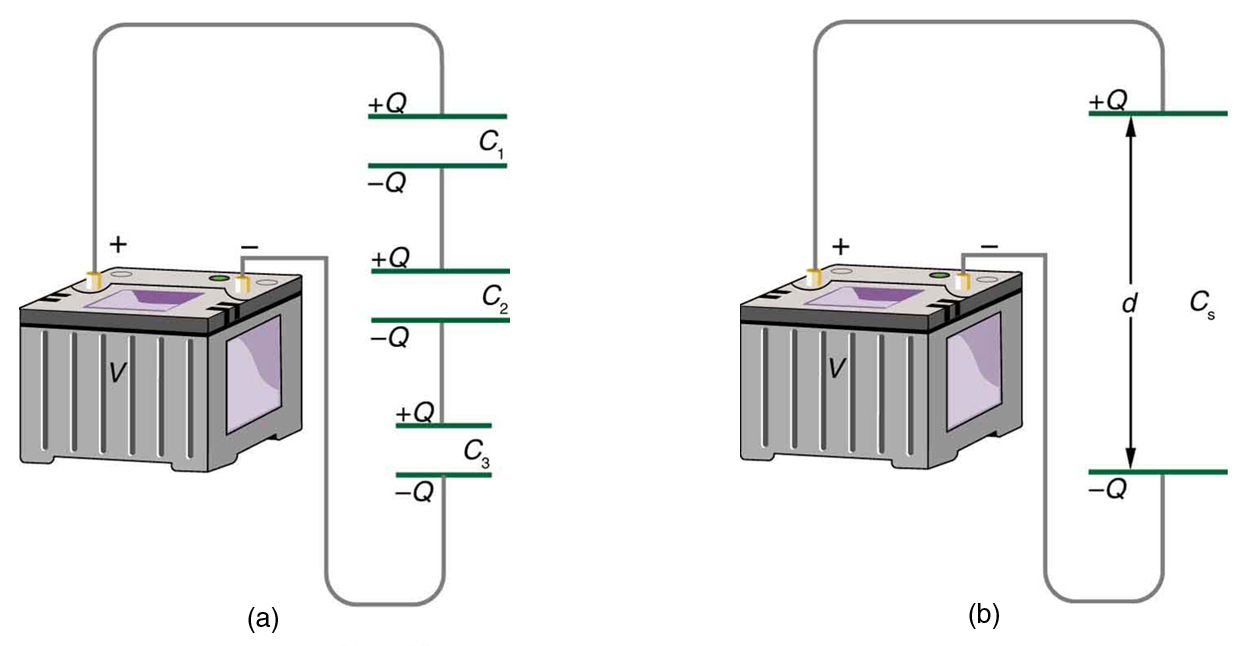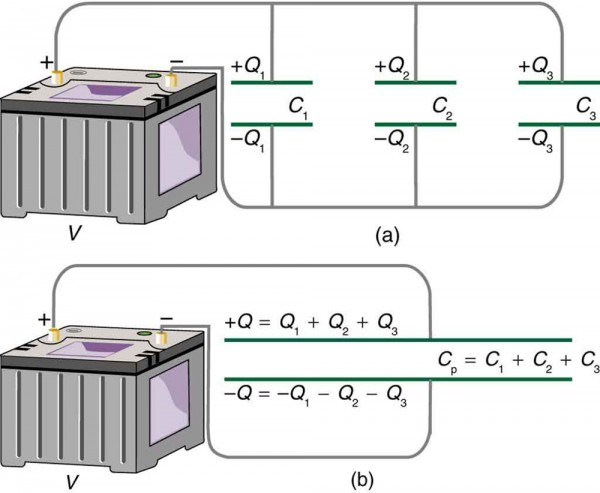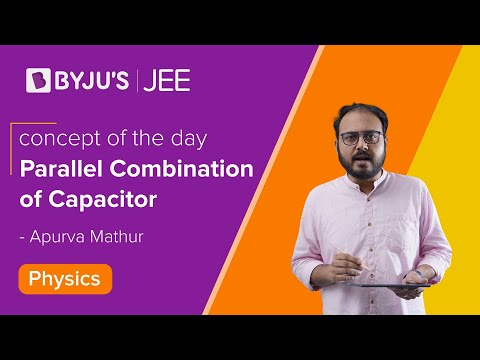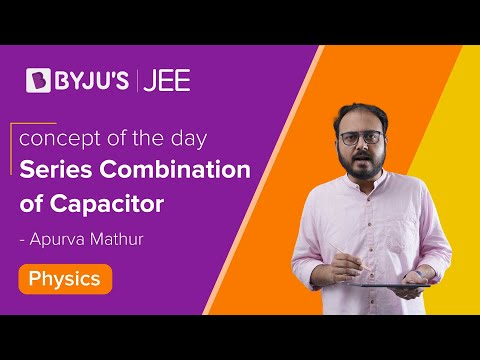# Capacitors in Parallel Questions

## Connection of Capacitors

The combination of capacitors includes a battery connected to it that applies a potential difference (V) and charges the plates (Q). The equivalent capacitance of the combination between two points to be defined as

C=\frac{Q}{V}C=

VQ

​Normally there are two capacitor combinations that are in practice – Parallel combination and Series combination

## Capacitors in Parallel

The figure given below shows a parallel connection of three capacitors with an applied voltage. Therefore, to calculate the overall capacitance that is, Cp) it is required to first note down that the voltage across each capacitor is V, since they are connected directly to it through a conductor. Thus, the capacitors have the same charges on them and the overall charge Q will be the sum of the individual charges that is Q = Q1 + Q2 + Q3.To understand the Capacitors in parallel, consider the figure (a) in which the capacitors are connected in parallel but, it looks like each capacitor is directly connected to the voltage source just as if it were all alone, and therefore, the sum of the individual capacitances in parallel will be the overall capacitance.

Figure (b) shows that the equivalent capacitor has a greater area of plate, which means that as compared to the individual capacitors, the equivalent capacitor can hold more charge.

By means of the relationship Q = CV,

the overall charge is Q = CpV,

and the individual charges will be Q1 = C1V, Q2 = C2V, and Q3 = C3V. Therefore,

CpV = C1V + C2V + C3V.

Eliminating V from the equation, the overall capacitance in parallel equation can be obtained as,

Cp: Cp = C1 + C2 + C3 + ….

Hence, the overall capacitance in parallel is just the sum of the individual capacitances.

## Capacitance in Series

To understand the capacitors connected in series, consider the figure given below which shows three capacitors connected in series with a voltage applied to the circuit. The capacitance of the combination is associated with the voltage and charge, which is given by C=Q/V for any capacitor.Figure (a) shows that when the voltage V is applied in the combination, the opposite charges of magnitude Q flow to either side of the initially uncharged combination of capacitors.

On each plate, the magnitude of the charge is Q and the equivalent capacitor has a larger plate separation d. The overall capacitance produced by a series connection is less than that of any of the individual capacitors.

The expression for the overall capacitance by considering the voltage across each capacitor can be calculated by solving

C=Q/V where V gives V=Q/C

Therefore, the voltages across the individual capacitors will be-

V1=Q/C1, V2=Q/C2, and V3=Q/C3

Hence, the overall voltage will be the sum of the individual voltages.

That is, V = V1 + V2 +V3.

Now, to calculate the overall capacitance CS for series combination capacitance, consider-

V = Q/CS = V1+V2+V3

Putting the value of V, the expressions for V1, V2, and V3, we will get

Q/CS = Q/C1+Q/C2+Q/C3

After cancelling the Qs, from the above equation, we get the overall capacitance in series CS that is,

1/CS =1/C1+1/C2+1/C3+…

This expression is valid for ‘n’ number of capacitors connected in series.

### Important Questions on Capacitors in Parallel

1) Calculate the overall capacitance when 3 capacitors of magnitude 1F, 4F and 6F are connected in parallel.

a) 11F

b) 12F

c) 34/24F

d) 25F

Correct Option: (a)

Explanation: When capacitors are connected in parallel, the overall capacitance C=C1+C2+C3. Therefore, C = 1+4+6 = 11F

2) Compute the voltage across AB when overall charge is stored in the combination 15C, where 3 capacitors of magnitude 5F, 6F and 4F are connected in parallel across AB.

a) 1V

b) 2.5V

c) 3V

d) 4V

Correct Option: (a)

Explanation: When capacitors are connected in parallel the overall capacitance C=C1+C2+C3. Therefore, C = 5+6+4 = 15F. Now to calculate the voltage, V = Q/C therefore, V = 15/15 = 1V

3) When capacitors K1, K2 and K3 are associated in parallel, what will be the overall capacitance of all the 3 capacitors?

a) K1/(K2-K3)

b) K1+K2+K3

c) K1/(K2+K3)

d) 1/K1+1/K2+1/K3

Correct Option: (b)

Explanation: The overall capacitance is equal to the sum of the capacitance of each of the capacitors, when connected in parallel. Hence, overall=K1+K2+K3.

4) Two capacitors of capacitance 2F and 4F are connected in parallel across the voltage of 100V. Calculate the charge across the capacitor of capacitance 4F.

a) 200C

b) 300C

c) 400C

d) 500C

Correct Option: (C)

Explanation: Since capacitors are connected in parallel, therefore, the voltage across each capacitor will be same and it does not get divided, hence, Q = CV which means Q = 4 *100 = 400C

5) The overall capacitance is always_____than the individual capacitance values, when capacitors are allied in parallel.

a) Lesser

b) Greater

c) Equal to

d) Cannot be determined

Correct Option: (b)

Explanation: The overall capacitance is equal to the sum of the capacitance of each of the capacitors, when connected in parallel. Hence, overall=C1+C2+C3. Therefore, the overall capacitance is greater than the individual capacitance values because it is the sum of all the capacitance values.

6) The effective plate area will_____when capacitors are connected in parallel.

a) Increase

b) Decrease

c) Remain the same

d) Become zero

Correct Option: (a)

Explanation: The top plates of each of the capacitors are connected together when capacitors are connected in parallel, but the plates at bottom are connected to each other. Due to this the top and bottom plate area is effectively increased.

7) Calculate the corresponding capacitance when two capacitors having capacitance value 6F, three capacitors having capacitance value 3F, and 4 capacitors having capacitance value 2F are connected in parallel.

a) 20F

b) 29F

c) 16F

d) 40F

Correct Option: (b)

Explanation: When capacitors are linked in parallel, the overall capacitance is equal to the sum of the capacitance of each of the capacitors, when connected in parallel. Hence overall=C1+C2+C3+……. +Cn Hence, Coverall=6+6+3+3+3+2+2+2+2=29F.

8) Calculate the overall charge of the system when 3 capacitors of capacitance 1F, 3F and 5F are connected in parallel across the voltage of 100V.

a) 200C

b) 300C

c) 900C

d) 500C

Correct Option: (C)

Explanation: The equivalent capacitance when capacitors are connected in parallel is the sum of all the capacitors=1+3+5=9F. Q = CV = 9*100 = 900C

9) N capacitors having capacitance C1 are connected in parallel, calculate the equivalent capacitance.

a) C1

b) C1/N

c) C1N

d) N/ C1

Correct Option: (c)

Explanation: The overall capacitance is equal to the sum of the capacitance of each of the capacitors, when connected in parallel. Hence overall= C11+ C12+ C13.……. N times.

Coverall= C1N

10) How to arrange three capacitors in a circuit to get high energy on the same potential?

a) Two in parallel on in series

b) All three in series

c) All three in parallel

d) None of these

Correct Option: (c)

Explanation: Since, the energy stored in a capacitor is directly proportional to the equivalent capacitance, hence the equivalent capacitance will be more when the capacitors are connected in parallel because in parallel arrangement the voltage across each capacitor will be the same.

### Practice Questions

1. Differentiate between parallel and series combinations in capacitors.
2. What is a capacitor?
3. Explain how energy is stored in a capacitor.
4. What will be the equivalent capacitance when two capacitors of capacitance C1 = 5 μ F and C2 = 2 μ F are connected in series across a cell of emf 20 V?